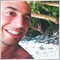# Help code this line: Close[i] > ‘x’ number of bars please363

I’m trying to code an indicator that displays an arrow if the close of candle [i] is > the close of a ‘specified’ number of previous candles (ie if it closes above the previous number of specified candles) but I can’t find my way out of this. Kindly help community.

```int OnInit()
{
//--- indicator buffers mapping
SetIndexBuffer(0, MA_HIGH);
PlotIndexSetString(0, PLOT_LABEL, " MA High");

SetIndexBuffer(1, MA_TP);
PlotIndexSetString(1, PLOT_LABEL, "MA TP");

SetIndexBuffer(2, MA_LOW);
PlotIndexSetString(2, PLOT_LABEL, "MA  Low");

SetIndexBuffer(3, up);
PlotIndexSetString(3, PLOT_LABEL, "Up");

SetIndexBuffer(4, dn);
PlotIndexSetString(4, PLOT_LABEL, "Dn");

PlotIndexSetInteger(0, PLOT_LINE_COLOR, clrLimeGreen);
PlotIndexSetInteger(0,PLOT_LINE_WIDTH,1);
PlotIndexSetInteger(1, PLOT_LINE_COLOR, clrRed);
PlotIndexSetInteger(1,PLOT_LINE_WIDTH,1);
PlotIndexSetInteger(2, PLOT_LINE_COLOR, clrBlue);
PlotIndexSetInteger(2,PLOT_LINE_WIDTH,1);

PlotIndexSetInteger(3, PLOT_ARROW, 241);
PlotIndexSetInteger(3, PLOT_ARROW_SHIFT, +10);
PlotIndexSetInteger(4, PLOT_ARROW, 242);
PlotIndexSetInteger(4, PLOT_ARROW_SHIFT, -10);

ArraySetAsSeries(up,true);
ArraySetAsSeries(dn,true);

MA_HIGH_handle=iMA(_Symbol,MA_H_TF,_MA_H_Period,_MA_H_Shift,_MA_H_Method,_MA_H_Price);
MA_TP_handle=iMA(_Symbol,MA_TP_TF,_MA_TP_Period,_MA_TP_Shift,_MA_TP_Method,_MA_TP_Price);
MA_LOW_handle=iMA(_Symbol,MA_L_TF,_MA_L_Period,_MA_L_Shift,_MA_L_Method,_MA_L_Price);
hrsi = iRSI(NULL, RsiTF, RsiPeriod, RsiApplied);

//-------------------------------------------------------------
return(INIT_SUCCEEDED);
}

void OnDeinit(const int reason)
{
//------------------------------------------------------------------
//------------------------------------------------------------------
}

//+------------------------------------------------------------------+
//| Custom indicator iteration function                              |
//+------------------------------------------------------------------+
int OnCalculate(const int rates_total,
const int prev_calculated,
const datetime &time[],
const double &open[],
const double &high[],
const double &low[],
const double &close[],
const long &tick_volume[],
const long &volume[],
{
//----------------------------------------------------------------------+
ArraySetAsSeries(open, true); ArraySetAsSeries(close, true);
ArraySetAsSeries(high, true); ArraySetAsSeries(low, true);
ArraySetAsSeries(time, true);
//----------------------------------------------------------------------+

CopyBuffer(MA_HIGH_handle,0,0,Bars(Symbol(),Period()),MA_HIGH);
ArraySetAsSeries(MA_HIGH,true);

CopyBuffer(MA_TP_handle,0,0,Bars(Symbol(),Period()),MA_TP);
ArraySetAsSeries(MA_TP,true);

CopyBuffer(MA_LOW_handle,0,0,Bars(Symbol(),Period()),MA_LOW);
ArraySetAsSeries(MA_LOW,true);

double HighestCandle;
double High[];
ArraySetAsSeries(High,true);
CopyHigh(_Symbol,_Period,0,11,High);
HighestCandle=ArrayMaximum(High,0,11);

double LowestCandle;
double Low[];
ArraySetAsSeries(Low,true);
CopyLow(_Symbol,_Period,0,11,Low);
LowestCandle=ArrayMinimum(Low,2,11);

double LowerCloseCandle;
double Close[];
ArraySetAsSeries(Close,true);
CopyClose(_Symbol,_Period,0,11,Close);
LowerCloseCandle=ArrayMinimum(Close,0,11);

//---------------------------------------------------------------------+
int limit = rates_total-prev_calculated;
if (prev_calculated==0) limit = rates_total-1;
for (int i = limit; i >= 0; i--)

{
up[i] = EMPTY_VALUE; dn[i] = EMPTY_VALUE;
if (MathAbs(close[i]-open[i]))
if (MathAbs(close[i]-open[i])>=Candle1BodySize*_Point )

{

if (close[i]>open[i]&&close[i]>MA_TP[i]&&low[i]<MA_HIGH[i])
up[i] = low[i];

if (close[i]<open[i]&&close[i]<MA_LOW[i]&&high[i]>MA_LOW[i]&&close[i]>=LowerCloseCandle)

dn[i] = high[i];

}
}```5082

Write your code and your try and maybe someone can be gentle to fix it for you.363

Fabio Cavalloni:

Write your code and your try and maybe someone can be gentle to fix it for you.5082

You wrote rules to create arrows when close[i] is above MA_TP and low[i] is below MA_HIGH.

It's totally different from the question you asked for (displays an arrow if the close of candle [i] is > the close of a ‘specified’ number of previous candles)363

Fabio Cavalloni:

You wrote rules to create arrows when close[i] is above MA_TP and low[i] is below MA_HIGH.

It's totally different from the question you asked for (displays an arrow if the close of candle [i] is > the close of a ‘specified’ number of previous candles)

Yes I  want to add this new rule(displays an arrow if the close of candle [i] is > the close of a ‘specified’ number of previous candles) to the already existing one(close[i] is above MA_TP and low[i] is below MA_HIGH). I tried using values from the High[] and Close [] array but didn't work so I deleted those lines of codes508220418

1. ```double HighestCandle;
HighestCandle=ArrayMaximum(High,0,11);```
Perhaps you should read the manual. The function does not return a double.

2. `     if (close[i]<open[i]&&close[i]<MA_LOW[i]&&high[i]>MA_LOW[i]&&close[i]>=LowerCloseCandle)`
You are looping through all candles, but LowerCloseCandle is only about the latest 11, instead of the eleven related to i.
How to do your lookbacks correctly.363

William Roeder:
1. Perhaps you should read the manual. The function does not return a double.

2. You are looping through all candles, but LowerCloseCandle is only about the latest 11, instead of the eleven related to i.
How to do your lookbacks correctly.

Thanks for your input sir, I have read the attached thread but still didn't  get it. All I want is to get the close of 'i' which is the lowest or highest among a specified number of previous consecutive candles. Any help with regards to the coding will be much appreciated. Thank you1092

Also you are copying the whole indicator buffers for every new tick. This is unnecessary and time consuming. Copy only limit values instead of using Bars(), and the ArraySetAsSeries on MA_HIGH/MA_LOW/MA_TP can be called once in OnInit. Then why don't you work with high directly instead of CopyHigh() to High?363

lippmaje:
Also you are copying the whole indicator buffers for every new tick. This is unnecessary and time consuming. Copy only limit values instead of using Bars(), and the ArraySetAsSeries on MA_HIGH/MA_LOW/MA_TP can be called once in OnInit. Then why don't you work with high directly instead of CopyHigh() to High?

Help me with the code please.1092

Ernest Gyamfi:

Help me with the code please.

```for (int i = limit; i >= 0; i--)
{
int index=ArrayMaximum(high,i,11);
double highest=high[index]; // highest high of the 11 bars at i```